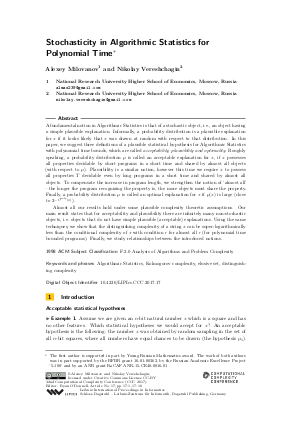Document# Stochasticity in Algorithmic Statistics for Polynomial Time

### Authors Alexey Milovanov, Nikolay Vereshchagin## File

LIPIcs.CCC.2017.17.pdf
• Filesize: 0.53 MB
• 18 pages

## Cite As

Alexey Milovanov and Nikolay Vereshchagin. Stochasticity in Algorithmic Statistics for Polynomial Time. In 32nd Computational Complexity Conference (CCC 2017). Leibniz International Proceedings in Informatics (LIPIcs), Volume 79, pp. 17:1-17:18, Schloss Dagstuhl - Leibniz-Zentrum für Informatik (2017)
https://doi.org/10.4230/LIPIcs.CCC.2017.17

## Abstract

A fundamental notion in Algorithmic Statistics is that of a stochastic object, i.e., an object having a simple plausible explanation. Informally, a probability distribution is a plausible explanation for x if it looks likely that x was drawn at random with respect to that distribution. In this paper, we suggest three definitions of a plausible statistical hypothesis for Algorithmic Statistics with polynomial time bounds, which are called acceptability, plausibility and optimality. Roughly speaking, a probability distribution m is called an acceptable explanation for x, if x possesses all properties decidable by short programs in a short time and shared by almost all objects (with respect to m). Plausibility is a similar notion, however this time we require x to possess all properties T decidable even by long programs in a short time and shared by almost all objects. To compensate the increase in program length, we strengthen the notion of `almost all' - the longer the program recognizing the property is, the more objects must share the property. Finally, a probability distribution m is called an optimal explanation for x if m(x) is large. Almost all our results hold under some plausible complexity theoretic assumptions. Our main result states that for acceptability and plausibility there are infinitely many non-stochastic objects, i.e. objects that do not have simple plausible (acceptable) explanations. Using the same techniques, we show that the distinguishing complexity of a string x can be super-logarithmically less than the conditional complexity of x with condition r for almost all r (for polynomial time bounded programs). Finally, we study relationships between the introduced notions.
##### Keywords
• Algorithmic Statistics
• Kolmogorov complexity
• elusive set
• distinguishing complexity

## Metrics

• Access Statistics
• Total Accesses (updated on a weekly basis)
0